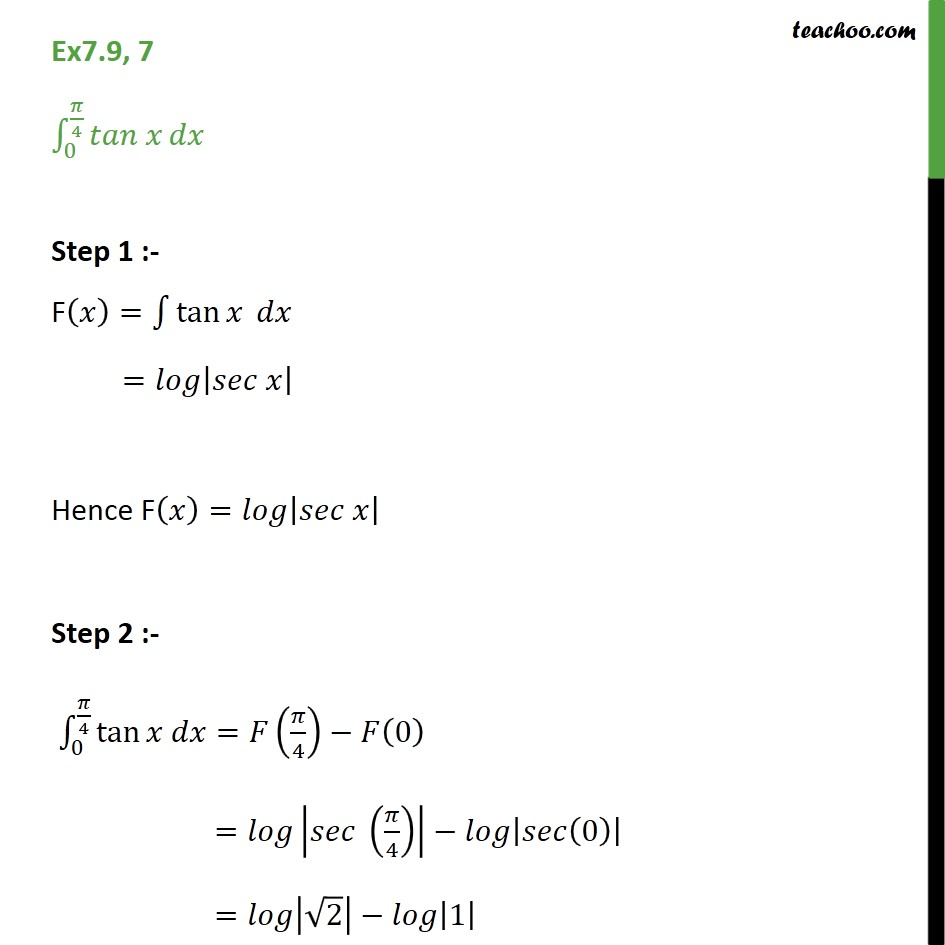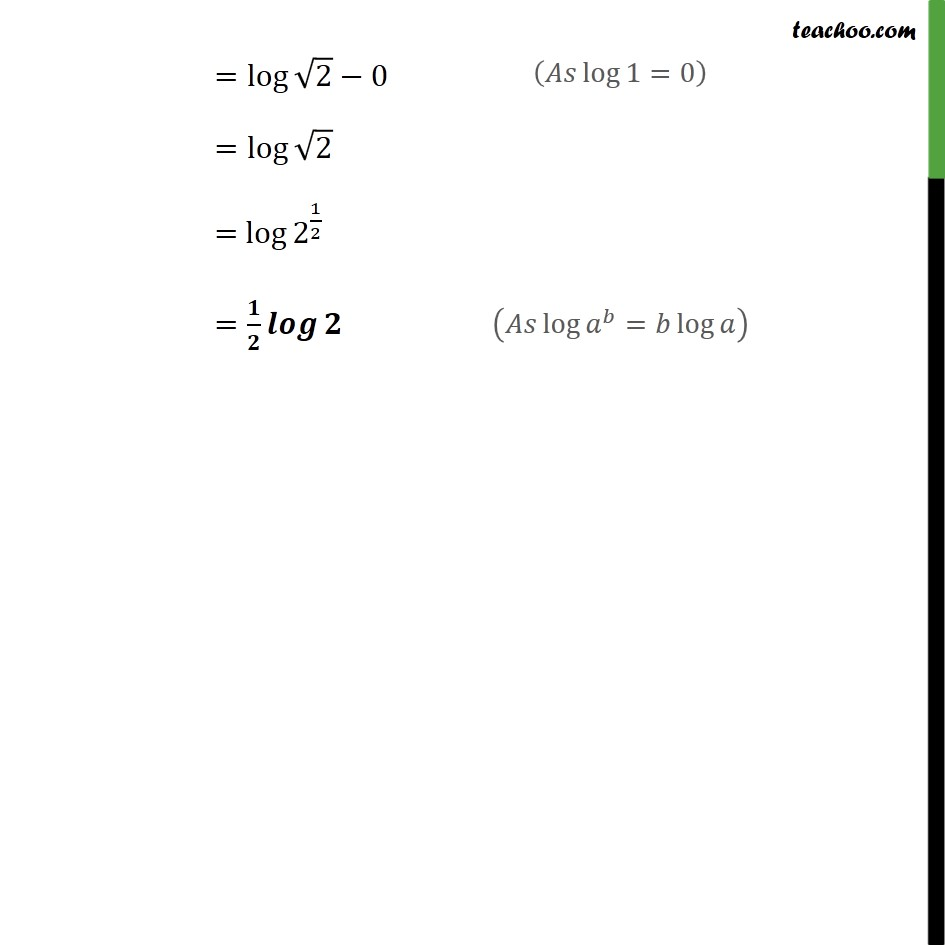Integration Full Chapter Explained - https://you.tube/Integration-Class-121. Chapter 7 Class 12 Integrals
2. Concept wise
3. Definite Integration - By Formulae

Transcript

Ex7.9, 7 0 4 Step 1 :- F = tan = Hence F = Step 2 :- 0 4 tan = 4 0 = 4 0 = 2 1 = log 2 0 = log 2 = log 2 1 2 =

Definite Integration - By Formulae